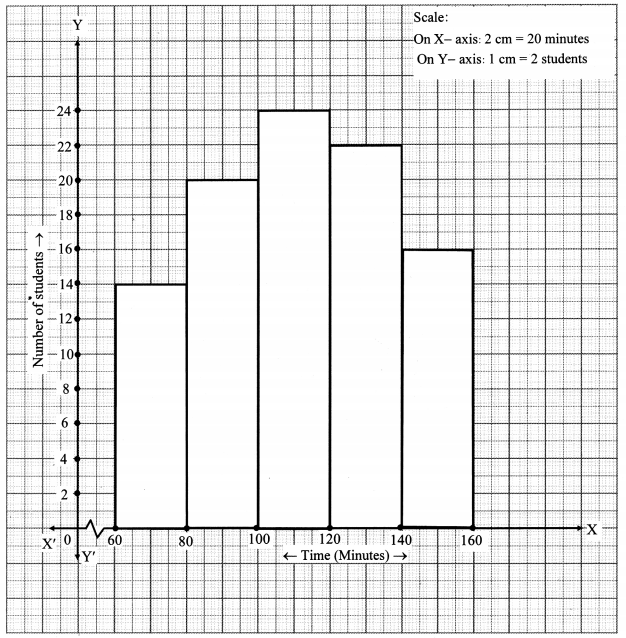# Maharashtra Board Class 10 Maths Solutions Chapter 6 Statistics Practice Set 6.4

## Maharashtra State Board Class 10 Maths Solutions Chapter 6 Statistics Practice Set 6.4

Question 1.
Draw a histogram of the following data.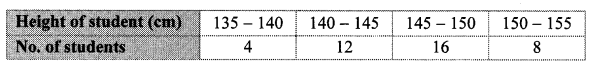Solution:Question 2.
The table below shows the yield of jowar per acre. Show the data by histogram.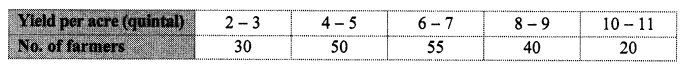Solution: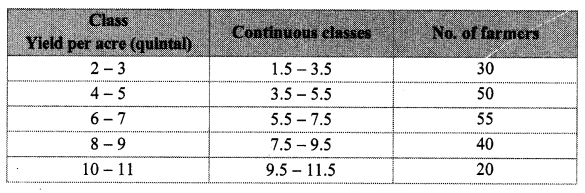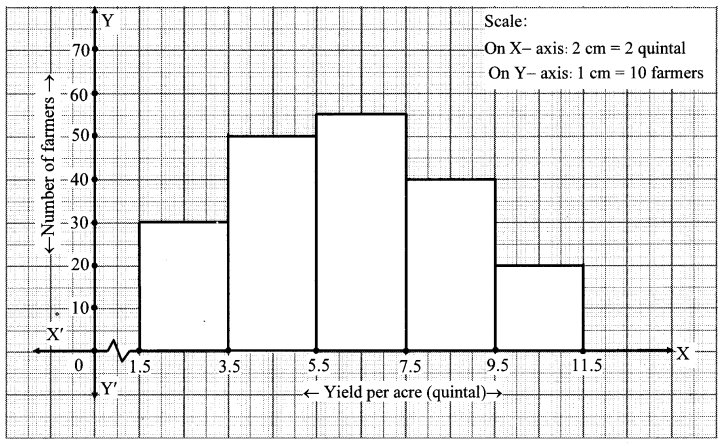Question 3.
In the following table, the investment made by 210 families is shown. Present it in the form of a histogram.Solution: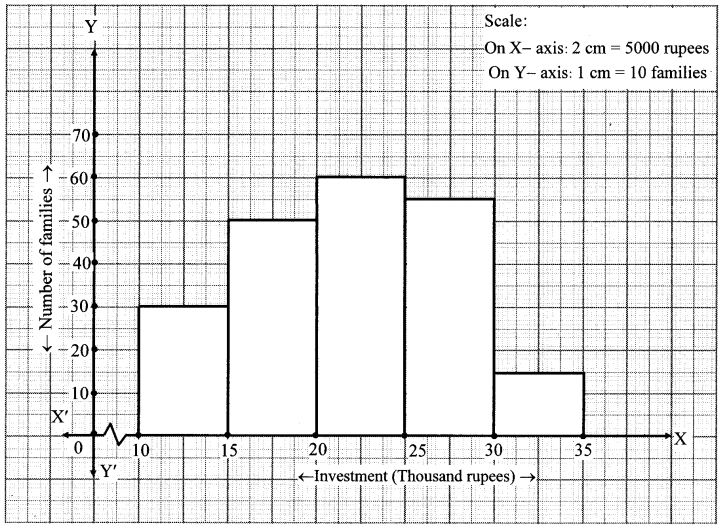Question 4.
Time allotted for the preparation of an examination by some students is shown in the table. Draw a histogram to show the information.Solution: